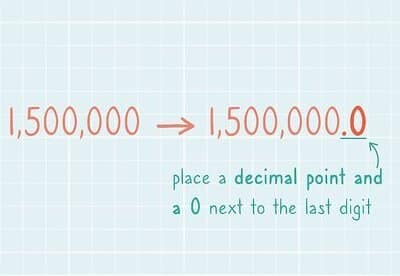Uh Oh! It seems you’re using an Ad blocker!

We always struggled to serve you with the best online calculations, thus, there's a humble request to either disable the AD blocker or go with premium plans to use the AD-Free version for calculators.

Or

Blog / Education / How to Write Numbers in Standard Form# How to Write Numbers in Standard Form

The standard form is also called scientific notation in the United States, a method used to express very large and small numbers in decimal form. It is used in both science and maths as shorthand rather than using the actual number every time you use it. Standard form makes calculation much easier rather than using a number that has multiple place values. Simply try a free standard form converter online that is designed to turn any number into its standard form within a fraction of seconds.

## What is The Standard Form?

A way of writing small and large numbers with one number before the decimal point and multiplied by a power of 10. For Example 290000 is a number which is written in standard form as 2.9 X 105. Use an online standard form calculator that helps to convert the number in standard form and also measure the Scientific E-Notation, Engineering notation, and Real numbers of the given number.

## Groups of Three:

The standard form refers to a form of notation in which digits are separated with the use of commas in groups of three. These groups of digits are known as periods.

For Example:

The number 321957756 is usually written in the form commas as 321,957,756. The first three numbers are called as one that appears when a number is expressed in standard form. This is even true if the first group contains just 1 or 2 digits. For example the first three digits of the number 75,236,149 would be 7, 5, and 2.

## Positive and Negative Exponents:

When a number is written as scientific notation, the exponent of the number tells that the term is larger or smaller. A positive exponent indicates the large number and negative number indicates about the small number which is between 0 and 1.

Examples:

The speed of light is about 299,792,458 meters/second. In standard form it can be written as 3.00 X 108 m/s. (remember that round the number 299 to 300 the digit at the fourth place is larger than 4).

The distance between the nucleus and electrons of the hydrogen atom is 0.00000000005291772 meters, so the standard form would be 5.29 X 10-11 meters. (In this case you don’t need to round up the value because the digit before 9 is less than 5).

Instead of performing complex calculations on numbers, give a try to the standard form converter that converts the number in standard form by inserting the decimal point in the given number.

## Arithmetic with Numbers in Standard Form:

The addition and subtraction of numbers in standard form is easy, as long as they have the same exponents. You can simply add or subtract the strings of the digits, if numbers have different exponents, and convert one of the numbers with the exponent of the other.

Example:

Add the number 4.45 X 1010 and 3.75 X 108. The first number is the same number as 445 X 108. Remember that, as the decimal point moves, the exponent will change. By adding them we get 447.75 X 108or - less accurately - 448 X 1010.

Try an online standard form calculator helps you to write the large and small numbers with a power of 10.

### Multiplication and Division:

When multiplying the number in standard form, you just need to multiply the strings and add the exponents. When you divide the by other numbers then you ought to apply the division operation on the number and subtract the exponent.

Examples
• Multiply 4.35 X 108 by 2.64 X 104
Convert to standard form:

It can be written as 11.49 X 1012.
• Divide 5.23 X 108by 1.24 X 104
After converting to standard form the answer will be written as 4.22. X 104.

## Final Words:

According to the mathematicians, standard form is indicated as the system of writing numbers which can be useful when working with large and small numbers. To provide help with math assignment, experts use standard form in various calculations such as measuring the speed of light, and distance between two planets that can be huge. However, in the past there is some uncertainty in measuring the distance of planets, but now, you can use an online standard form calculator to turn the large and small numbers into its standard form.### Jacquelin Smith

Last updated: June 27, 2023

Jacquelin Smith is an accomplished writer and a Researcher; She has done MS(Computer Science) and doing content writing for since 2019. She is an ardent writer and expert in Niches like Digital Marketing, Social media, Mathematics, etc. She is an expert writer and commentator at the same time.

Search